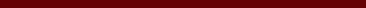### PELABELAN TOTAL TIDAK TERATUR TITIK GRAF UNIDENTIFIED FLYING OBJECT

Andi Daniah Pahrany, Nurdin Nurdin, Hasmawati Hasmawati

#### Abstract

For a graph G that have vertex set V and edge set E. A labelling f:VUE->{1,2,3,...,k} is called a vertex irregularity total k-labeling of G if for any two different vertices x and y in V have wt(x)\neq wt(y) where wt(x)=f(x)+∑_{yV(G)} f(xy). The smallest positive integer k such that G has a vertex irregular total k-labeling is called the total vertex irregularity strength of G  , denoted by tvs(G).

In this paper, we determined the total vertex irregularity strength of Unidentified Flying Object Graph, tvs(U_(m,n}). The result in this paper as follows:

tvs(U_{m,n})=(3n+1)/2 for 3<=m<=3((n+1)/2), n>=3, and

tvs(U_{m,n})=(3n+m)/3 for m>3((n+1)/2), n>=3.

#### Full Text:

FULL PDF

DOI: http://dx.doi.org/10.17977/um055v3i22022p15-20

### Refbacks

• There are currently no refbacks.

Copyright (c) 2022 Andi Daniah Pahrany, Nurdin Nurdin, Hasmawati Hasmawati